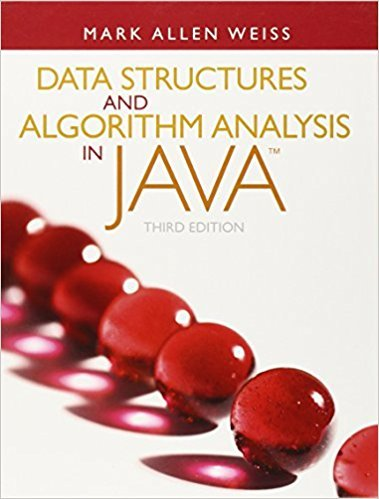×
Get Full Access to Data Structures And Algorithm Analysis In Java - 3 Edition - Chapter 12 - Problem 12.9
Get Full Access to Data Structures And Algorithm Analysis In Java - 3 Edition - Chapter 12 - Problem 12.9

×

# Once the suffix array is constructed, the short routine shown in Figure 12.50 canbeISBN: 9780132576277 316

## Solution for problem 12.9 Chapter 12

Data Structures and Algorithm Analysis in Java | 3rd Edition

• Textbook Solutions
• 2901 Step-by-step solutions solved by professors and subject experts
• Get 24/7 help from StudySoup virtual teaching assistantsData Structures and Algorithm Analysis in Java | 3rd Edition

4 5 1 417 Reviews
14
5
Problem 12.9

Once the suffix array is constructed, the short routine shown in Figure 12.50 canbe invoked from Figure 12.32 to create the longest common prefix array.a. In the code, what does rank[i] represent?b. Suppose that LCP[rank[i] ] = h. Show that LCP[rank[i+1] ] h 1.c. Show that the algorithm in Figure 12.50 correctly computes the LCP array.d. Prove that the algorithm in Figure 12.50 runs in linear time.1 /*2 * Create the LCP array from the suffix array3 * @param s the input array populated from 0..N-1, with available pos N4 * @param sa the already-computed suffix array 0..N-15 * @param LCP the resulting LCP array 0..N-16 */7 public static void makeLCPArray( int [ ] s, int [ ] sa, int [ ] LCP )8 {9 int N = sa.length;10 int [ ] rank = new int[ N ];1112 s[ N ] = -1;13 for( int i = 0; i < N; i++ )14 rank[ sa[ i ] ] = i;1516 int h = 0;17 for( int i = 0; i < N; i++ )18 if( rank[ i ]>0)19 {20 int j = sa[ rank[ i ] - 1 ];2122 while( s[ i + h ] == s[ j + h ] )23 h++;2425 LCP[ rank[ i ] ] = h;26 if( h > 0 )27 h--;28 }29 }Figure 12.50 LCP array construction from suffix array

Step-by-Step Solution:
Step 1 of 3

Week 5 2/8/16  energy (ch.3) o great idea: the many different forms of energy are interchangeable, and the total amount of energy in an isolated system is conserved o the great chain of energy  scientifically speaking  energy related to force  Work o force over distance o formula: W = Fd o Units: joules  Energy o Ability to do work  Power o Rate at which work is done o Equation: P = W/t or (E – f)/ti o Units: watts  Basic ideas  Energy can be provided/stored in various forms  Food is one way to store energy. Our bodies store energy in the form of fat, which can be converted back in terms of low availability of food  Other units for energy  A calorie is the energy needed to increase the temperature of 1 gram of water be 1 degree Celsius (4.2 J)  A kilocalorie (aka food calorie) is the energy needed to increase the temperature of 1kg of water by 1 degree Celsius, this is exactly 1000 small calories

Step 2 of 3

Step 3 of 3

##### ISBN: 9780132576277

Data Structures and Algorithm Analysis in Java was written by and is associated to the ISBN: 9780132576277. The answer to “Once the suffix array is constructed, the short routine shown in Figure 12.50 canbe invoked from Figure 12.32 to create the longest common prefix array.a. In the code, what does rank[i] represent?b. Suppose that LCP[rank[i] ] = h. Show that LCP[rank[i+1] ] h 1.c. Show that the algorithm in Figure 12.50 correctly computes the LCP array.d. Prove that the algorithm in Figure 12.50 runs in linear time.1 /*2 * Create the LCP array from the suffix array3 * @param s the input array populated from 0..N-1, with available pos N4 * @param sa the already-computed suffix array 0..N-15 * @param LCP the resulting LCP array 0..N-16 */7 public static void makeLCPArray( int [ ] s, int [ ] sa, int [ ] LCP )8 {9 int N = sa.length;10 int [ ] rank = new int[ N ];1112 s[ N ] = -1;13 for( int i = 0; i < N; i++ )14 rank[ sa[ i ] ] = i;1516 int h = 0;17 for( int i = 0; i < N; i++ )18 if( rank[ i ]>0)19 {20 int j = sa[ rank[ i ] - 1 ];2122 while( s[ i + h ] == s[ j + h ] )23 h++;2425 LCP[ rank[ i ] ] = h;26 if( h > 0 )27 h--;28 }29 }Figure 12.50 LCP array construction from suffix array” is broken down into a number of easy to follow steps, and 225 words. Since the solution to 12.9 from 12 chapter was answered, more than 304 students have viewed the full step-by-step answer. This textbook survival guide was created for the textbook: Data Structures and Algorithm Analysis in Java, edition: 3. The full step-by-step solution to problem: 12.9 from chapter: 12 was answered by , our top Science solution expert on 03/02/18, 06:05PM. This full solution covers the following key subjects: . This expansive textbook survival guide covers 12 chapters, and 457 solutions.

## Discover and learn what students are asking

Calculus: Early Transcendental Functions : Integration Techniques, LHpitals Rule, and Improper Integrals
?Verify the reduction formula $$int \tan ^{n} x d x=\frac{1}{n-1} \tan ^{n-1} x-\int \tan ^{n-2} x d x$$

Calculus: Early Transcendental Functions : Differential Equations: Growth and Decay
?In Exercises 1-10, solve the differential equation. $$y^{\prime}=\frac{5 x}{y}$$

Unlock Textbook Solution